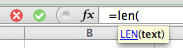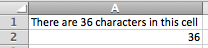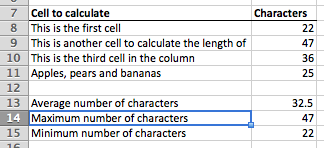# Count the number of characters in a cell in Excel

This lesson introduces the LEN() function, which allows you to calculate the number of characters in a cell. This formula is useful on its own, or can be combined with other text functions such as RIGHT(), LEFT(), MID() and FIND().

## LEN() function syntax

The LEN() function has the following syntax:

=LEN(text)

• Where text is the text value for which you want to calculate the number of characters, or a reference to a cell that contains a text value.
• Note that the LEN() function will count the number of characters in a cell regardless of whether the value in that cell is text or a number, or a combination of both.

## Example of the LEN() function in action

To use the LEN() function, follow these steps:

• Click on a cell and enter the function• Enter the reference to a cell that contains the text for which you want to calculate the length:• Once you have finished, press Enter to complete the formula and see the result:• You can use the LEN() function to calculate the length of values in a column of cells. This example also uses the AVERAGE(), MAX() and MIN() functions: